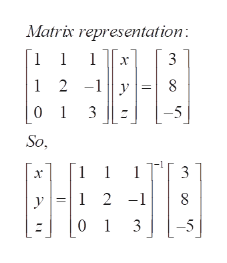# How do I solve the simultaneous following equations using matrix algebra?x + y + z = 3x + 2y - z = 8y +3z = -5

Question

How do I solve the simultaneous following equations using matrix algebra?

x + y + z = 3

x + 2y - z = 8

y +3z = -5

check_circleExpert Solution
Step 1

To apply matrix method to solve the given simultaneous equations

Step 2

If the system is represented by the matrix equation AV= b, then the solution vector V= A^(-1)b, where A^(-1) is the inverse...help_outlineImage TranscriptioncloseMatrix representation: | 1 2 -1y 8 01 3 So ELE S789 1 3 1 2-1 y 1 3 fullscreen

### Want to see the full answer?

See Solution

#### Want to see this answer and more?

Solutions are written by subject experts who are available 24/7. Questions are typically answered within 1 hour*

See Solution
*Response times may vary by subject and question
Tagged in

### Math